# Axisymmetric Instabilities to Avoid

When constructing rotating equilibrium configurations that obey a barotropic equation of state, keep in mind that certain physical variable profiles should be avoided because they will lead to structures that are unstable toward the dynamical development of shape-distorting or convective-type motions. Here are a few well-known examples.

## Rayleigh-Taylor Instability

Referencing both p. 101 of [ Shu92 ], and volume I, p. 410 of [ P00 ], a Rayleigh-Taylor instability is a bouyancy-driven instability that arises when a heavy fluid rests on top of a light fluid in an effective gravitational field,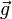$~\vec{g}$. In the simplest case of spherically symmetric, self-gravitating configurations, the condition for stability against a Rayleigh-Taylor instability may be written as,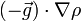$~(- \vec{g} ) \cdot \nabla\rho$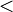$~<$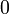$~0$ [stable] ,

that is to say, the mass density must decrease outward. In an expanded discussion, [ P00 ] — see pp. 410 - 413 — derives a dispersion relation that describes the development of the Rayleigh-Taylor instability in a plane-parallel fluid layer that initially contains a discontinuous jump/drop in the density.

In addition, [ P00 ] — see pp. 413 - 416 — and [ Shu92 ] — see pp. 101 - 105 — both discuss circumstances that can give rise to the so-called Kelvin-Helmholtz instability. It is another common two-fluid instability, but one that depends on the existence of transverse velocity flows and that is independent of the gravitational field.

## Poincaré-Wavre Theorem

As [ T78 ] points out — see his pp. 78 - 81 — Poincaré and Wavre were the first to, effectively, prove the following theorem:

 For rotating, self-gravitating configurations "any of the following statements implies the three others:   (i) the angular velocity is a constant over cylinders centered about the axis of rotation, (ii) the effective gravity can be derived from a potential, (iii) the effective gravity is normal to the isopycnic surfaces, (iv) the isobaric- and isopycnic-surfaces coincide."

Among other things, this implies that for rotating barotropic configurations not only is the equation of state given by a function of the form,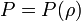$~P = P(\rho)$, but it must also be true that,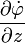$~\frac{\partial \dot\varphi}{\partial z}$$~=$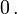$~0 \, .$ [ T78 ], §4.3, Eq. (30)

NOTE: We should investigate how this theorem comes into play in the context of our accompanying discussion of Type 1 Riemann ellipsoids. These are equilibrium triaxial, uniform-density configurations in which the system's internal vorticity vector does not align with the tumble-axis of the ellipsoid and, therefore apparently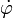$~\dot\varphi$ is not independent of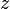$~z$.

## Høiland Criterion

 "For an incompressible liquid contained between concentric cylinders and rotating with angular velocity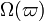$~\Omega(\varpi)$, Rayleigh's criterion — that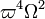$~\varpi^4 \Omega^2$ increase outwards — is necessary and sufficient for stability to axisymmetric disturbances. In a star rotating with angular velocity$~\Omega(\varpi)$ if we continue to restrict attention to axisymmetric disturbances, this criterion must be modified by buoyancy effects; that is, some combination of the Rayleigh and Schwarzschild criteria should obtain. Such a combination has been found, for example, by Høiland (1941) (see also Ledoux's Chapter 10, pp. 499-574 of Stellar Structure (1965), and indicates, as one would expect, that a stable stratification of angular velocity exerts a stabilizing influence on an unstable distribution of temperature, and vice versa. The combined criterion has not been placed on the solid analytical foundation of its two component criteria, however." — Drawn from p. 475 of N. R. Lebovitz (1967), ARAA, 5, 465

As is stated on p. 166 of [ T78 ], in rotating barotropic configurations, axisymmetric stability requires the simultaneous satisfaction of the following pair of conditions: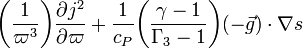$~\biggl(\frac{1}{\varpi^3} \biggr) \frac{\partial j^2}{\partial \varpi} + \frac{1}{c_P} \biggl( \frac{\gamma - 1}{\Gamma_3 - 1}\biggr) (- \vec{g} ) \cdot \nabla s$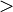$~>$$~0$ [stable] ; [ T78 ], §7.3, Eq. (41) see also[ KW94 ], §43.2, Eq. (43.22)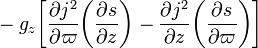$~-g_z \biggl[ \frac{\partial j^2}{\partial \varpi} \biggl(\frac{\partial s}{\partial z} \biggr) - \frac{\partial j^2}{\partial z} \biggl(\frac{\partial s}{\partial \varpi} \biggr)\biggr]$$~>$$~0$ [stable] . [ T78 ], §7.3, Eq. (42) see also[ KW94 ], §43.2, Eq. (43.23)

where,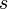$~s$, is the local specific entropy, and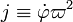$~j \equiv \dot\varphi \varpi^2$, is the local specific angular momentum of the fluid. According to [ T78 ] — see p. 168 — this pair of mathematically expressed conditions has the following meaning:

 "A baroclinic star in permanent rotation is dynamically stable with respect to axisymmetric motions if and only if the two following conditions are satisfied:   (i) the entropy per unit mass,$~s$, never decreases outward, and (ii) on each surface$~s$ = constant, the angular momentum per unit mass,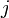$~j$, increases as we move from the poles to the equator."

### Schwarzschild Criterion

In the case of nonrotating equilibrium configurations, the Høiland Criterion reduces to the Schwarzschild criterion. That is, thermal convection arises when the condition,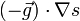$~(- \vec{g} ) \cdot \nabla s$$~>$$~0$ [stable] , [ T78 ], §7.3, Eq. (43) see also[ KW94 ], §6.1, Eq. (6.13) … or … pp. 93 - 98 of [ Shu92 ]

is violated. This means that, in order for a spherical system to be stable against dynamical convective motions, the specific entropy must increase outward.

### Solberg/Rayleigh Criterion

In the case of an homentropic equilibrium configuration, the Høiland Criterion reduces to the Solberg criterion. That is, an axisymmetric exchange of fluid "rings" will occur on a dynamical time scale if the condition,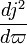$~\frac{dj^2}{d\varpi}$$~>$$~0$ [stable] , [ T78 ], §7.3, Eq. (44) see also[ KW94 ], §43.2, Eq. (43.18) … or … pp. 98 - 101 of [ Shu92 ]

is violated. This means that, for stability, the specific angular momentum must necessarily increase outward. As [ T78 ] points out, this "Solberg criterion generalizes to homentropic bodies the well-known Rayleigh (1917) criterion for an inviscid, incompressible fluid."

Here are some recommendations to keep in mind as you attempt to construct equilibrium models of self-gravitating astrophysical fluids.

Rayleigh-Taylor instability:  In order to avoid constructing configurations that are subject to the Rayleigh-Taylor instability, be sure that lower density material is never placed "beneath" higher density material. For example …

• When building a bipolytropic configuration, a value for the (imposed) discontinuous jump in the mean-molecular weight will need to be specified at the interface between the envelope and the core. If you choose a ratio,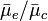$~{\bar\mu}_e/{\bar\mu}_c$, that is greater than unity, the resulting equilibrium model will exhibit a discontinuous density jump that makes the density higher at the base of the envelope than it is at the surface of the core. The core/envelope interface of this configuration will be unstable to the Rayleigh-Taylor instability, but you won't know that until and unless you examine the hydrodynamic stability of the configuration.

Schwarzschild criterion:  In order to avoid constructing configurations that violate the Schwarzschild criterion, be sure that the specific entropy of the fluid is uniform (marginally stable) or increases outward throughout the equilibrium structure, where the word "outward" is only meaningful when referenced against the direction that the effective gravity points. For example …

• Suppose that you build a spherically symmetric polytropic configuration whose structural index is,$~n$, but then you want to test the stability of the configuration assuming that compressions/expansions of individual fluid elements occur along adiabats for which the adiabatic index is,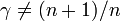$~\gamma \ne (n+1)/n$. Although we generally think of polytropes as being homentropic configurations, if$~\gamma \ne (n+1)/n$, then different fluid elements will, in practice, evolve along adiabats that are characterized by different values of the specific entropy; that is, throughout the equilibrium model,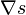$~\nabla s$ will not be zero. Whether the specific entropy increase (stable) or decreases (unstable) outward will depend on whether you select a value for the evolutionary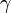$~\gamma$ that is greater than (stable) or less than (unstable)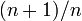$~(n+1)/n$.
• According to Woosley's class lecture notes,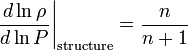$~\frac{d\ln \rho}{d\ln P}\biggr|_\mathrm{structure} = \frac{n}{n+1}$$~>$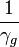$~\frac{1}{\gamma_g}$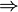$~\Rightarrow$      stable$~<$$~\frac{1}{\gamma_g}$$~\Rightarrow$      unstable

This is another way of expressing the same stability criterion for polytopes.

• Examples:   An n = 1 polytope is unstable toward convection if expansions (or contractions) occur along an adiabat with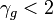$~\gamma_g < 2$. Alternatively, an n = 5 polytope is unstable toward convection if expansions (or contractions) occur along an adiabat with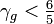$~\gamma_g < \tfrac{6}{5}$.